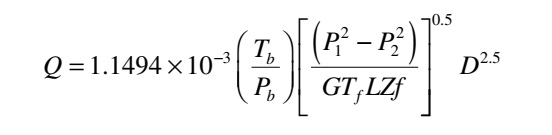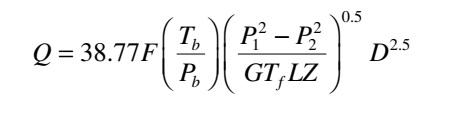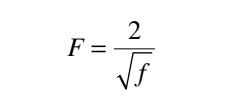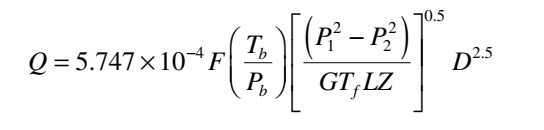General Gas Flow Equation:

The General Gas Flow equation, is also called the Fundamental Gas Flow equation, for the steady-state isothermal flow in a gas pipeline is the basic equation for relating the pressure drop with flow rate.The most common form of this equation in the U.S. Customary System (USCS) of units is given in terms of the pipe diameter, gas properties, pressures, temperatures, and flow rate as follows.Where,
Q = gas flow rate, measured at standard conditions, ft3/day (SCFD)
f = friction factor, dimensionless
Pb = base pressure, psia
Tb = base temperature, °R (460 + °F)
P1 = upstream pressure, psia
P2 = downstream pressure, psia
G = gas gravity (air = 1.00)
Tf = average gas flowing temperature, °R (460 + °F)
L = pipe segment length, mile
Z = gas compressibility factor at the flowing temperature, dimensionless
D = pipe inside diameter, inch

It must be noted that for the pipe segment from section 1 to section 2, the gas temperature Tf is assumed to be constant (isothermal flow).

In SI units, the General Flow equation is stated as follows:Where,
Q = gas flow rate, measured at standard conditions, m3/day
f = friction factor, dimensionless
Pb = base pressure, kPa
Tb= base temperature, K (273 + °C)
P1 = upstream pressure, kPa
P2 = downstream pressure, kPa
G = gas gravity (air = 1.00)
Tf= average gas flowing temperature, K (273 + °C)
L = pipe segment length, km
Z = gas compressibility factor at the flowing temperature, dimensionless
D = pipe inside diameter, mm

• Above flow equation relates the capacity (flow rate or throughput) of a pipe segment of length L, based on an upstream pressure of P1 and a downstream pressure of P2. It is assumed that there is no elevation difference between the upstream and downstream points; therefore, the pipe segment is horizontal.

• We see that for a pipe segment of length L and diameter D, the gas flow rate Q (at standard conditions) depends on several factors. Q depends on gas properties represented by the gravity G and the compressibility factor Z.

• If the gas gravity is increased (heavier gas), the flow rate will decrease. Similarly, as the compressibility factor Z increases, the flow rate will decrease. Also, as the gas flowing temperature Tf increases, throughput will decrease. Thus, the hotter the gas, the lower the flow rate will be. Therefore, to increase the flow rate, it helps to keep the gas temperature low. The impact of pipe length and inside diameter is also clear. As the pipe segment length increases for given pressure P1 and P2, the flow rate will decrease. On the other hand, the larger the diameter, the larger the flow rate will be.

• The term P12P22 represents the driving force that causes the flow rate from the upstream end to the downstream end. As the downstream pressure P2 is reduced, keeping the upstream pressure P1 constant, the flow rate will increase. It is obvious that when there is no flow rate, P1 is equal to P2. It is due to friction between the gas and pipe walls that the pressure drop (P1P2) occurs from the upstream point 1 to downstream point 2. The friction factor f depends on the internal condition of the pipe as well as the type of flow (laminar or turbulent).

Sometimes the General Flow equation is represented in terms of the transmission factor F instead of the friction factor f. This form of the equation is as follows in USCS Units.Where, the transmission factor F and friction factor f are related byThen General Flow equation in SI Units,